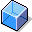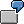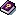TemplateDefinition

A template for the Formula-Controlled Calculation of primary costs and statistical key figures on cost centers and activity inputs on cost centers/activity.

Use

In a planning template, you can store Formulas, which the R/3 System evaluates. They are then used to calculate primary costs, statistical key figures, or activity inputs on the cost centers. Each planning template is assigned to an Environment. Among the factors you specify in the environment is whether a planning template is to be used for the planning of activity-independent or activity-dependent costs, statistical key figures, or activity inputs.

Structure

A planning template consists of rows and columns. You can identify the rows through a specified object type. These can be, for example, Calculation Rows, comment rows or rows for the reading of a cost element.

You can use the column parameters to specify the exact characteristic values of the row to be calculated. The following columns exist:

• Object types:

Specifies the characteristic value of the given row, for example, comment rows, calculation rows, resources, cost elements, statistical key figures, sub-templates.If you choose the "Cost element" type, you can store a formula for a cost element in this row. To calculate the value for the given cost element, the R/3 System takes planned values that you have used in the formula.

If you choose the "Calculation row" type, you can use another formula in this row to link previously defined rows.

• Name:

The name contains a description of the following rows:

• Object:

You can enter an object or go to a sub-template. The R/3 System calculates a value for the object in a formula that is yet to be defined.

• Cost formulas:
• Fixed costs

To calculate fixed costs, you can enter a formula in the CPI environment for the planning of activity-independent costs. See also: Formulas.

• Variable costs per activity unit

In the CPD environment for the planning of activity-dependent costs, you can enter a formula for the calculation of fixed, and variable, costs per activity unit.

• Quantity formula
• Fixed quantity

In the CPI environment for the planning of activity-independent costs and key figures, you can enter a formula for the calculation of fixed quantities of a cost element, statistical key figure, resource or the value of a calculation row. See also: Formulas.

• Variable costs per activity unit

In the CPD environment for the planning of activity-dependent costs and key figures, you can enter a formula for the calculation of fixed and variable quantities per activity unit of a cost element, statistical key figure or resource.

You enter a condition that controls the evaluation of the formula or sets the previously defined rows to active or inactive.

For more information, see the SAP Library under Activity-Based Costing underTemplate and Processing Templates.